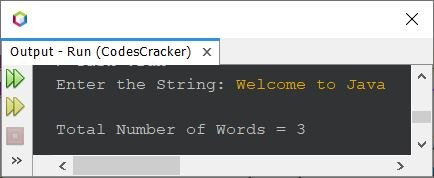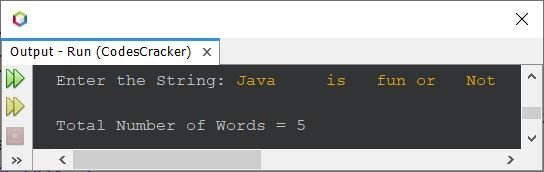# Java Program to Count Number of Words in a String

## Count Words in String - Basic Version

The question is, write a Java program to count the number of words in a string. The string must be entered by user at run-time of the program. The program given below is the answer:

```import java.util.Scanner;

public class CodesCracker
{
public static void main(String[] args)
{
String str;
int totalWords;
Scanner s = new Scanner(System.in);

System.out.print("Enter the String: ");
str = s.nextLine();

String words[] = str.split(" ");
totalWords = words.length;
System.out.println("\nTotal Number of Words = " +totalWords);
}
}```

Here is its sample run with user input, Welcome to Java as string to count total words available in it:In above program, the following statement:

`String words[] = str.split(" ");`

is used to split the string str by white spaces. That above statement can also be replaced with the statement given below:

`String words[] = str.split("\\s");`

## Count Words in String - Complete Version

The problem with previous program is, what if user enters a string in which there is/are single or multiple words separated with more than one white spaces. Therefore, in that case, we need to replace the above statement with:

`String words[] = str.split("\\s+");`

Here is the complete version of the program:

```import java.util.Scanner;

public class CodesCracker
{
public static void main(String[] args)
{
Scanner s = new Scanner(System.in);

System.out.print("Enter the String: ");
String str = s.nextLine();

String words[] = str.split("\\s+");
System.out.println("\nTotal Number of Words = " +words.length);
}
}```

The snapshot given below shows the sample run with a string input that contains some words separated with multiple white spaces:The same job of counting total number of words in a given string, can also be done using the following program:

```import java.util.Scanner;

public class CodesCracker
{
public static void main(String[] args)
{
String str;
int i, strLen, count=1;
Scanner s = new Scanner(System.in);

System.out.print("Enter the String: ");
str = s.nextLine();

strLen = str.length();
for(i=0; i<=(strLen-1); i++)
{
if(str.charAt(i) == ' ' && str.charAt(i+1)!=' ')
count++;
}

System.out.println("\nTotal Number of Words = " +count);
}
}```

You'll get the same output as of previous program.

Java Online Test

« Previous Program Next Program »